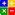# Ratio - Days Sales Outstanding (DSO)DAYS SALES OUTSTANDING (DSO):Online DSO Ratio Calculator

The 'Days Sales Outstanding' ratio shows both the average time it takes to turn the receivables into cash and the age, in terms of days, of a company's accounts receivable. The ratio is regarded as a test of 'efficiency' for a company. The effectiveness with which it converts its receivables into cash. This ratio is of particular importance to credit and collection associates. We will call this ratio Regular or Standard DSO.

BEST POSSIBLE DAYS SALES OUTSTANDING (Best Possible DSO)Online Best Possible DSO Ratio Calculator

Apart from the Regular DSO there is another measure called 'Best Possible DSO'. The 'Best Possible DSO', yields insight into delinquencies since it uses only the current portion of receivables. As a measurement, the closer the Regular DSO is to the Best Possible DSO, the closer the receivables are to the optimal level.

Best Possible DSO requires three pieces of information for its calculation:

•Current Receivable
•Total credit sales for the period analyzed
•The Number of days in the period analyzed

The formula:

Best Possible DSO = (Current Receivables/Total Credit Sales)  x Number of Days in the period that is being analyzed

The formula:

Regular DSO = (Total Accounts Receivables/Total Credit Sales) x Number of Days in the period that is being analyzed

Example:

Total Accounts Receivable = \$15,000
Current Accounts Receivable = \$4,000
Credit Sales made in a 30 days period = \$10,000

Regular DSO = (Total Accounts Receivables/Total Credit Sales) x Number of Days in the period that is being analyzed
Regular DSO = (15,000/10,000) x 30
Regular DSO = 45 Days
Interpretation: On an average it is taking 45 days to convert accounts receivable into Cash. That is to say if your Terms are Net 30 days then your customers on an average are taking an additional 15 days to pay their bills.

Best Possible DSO = (Current Receivables/Total Credit Sales) x Number of Days in the period that is being analyzed
Best Possible DSO = (4,000/10,000) x 30
Best Possible DSO = 12 Days
Interpretation: The current debtors are taking 12 days to pay